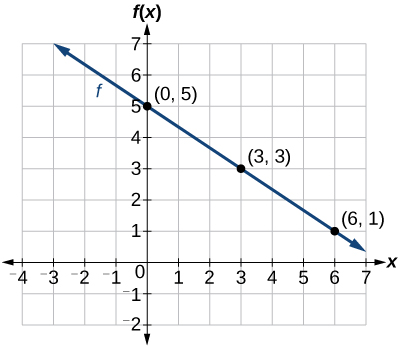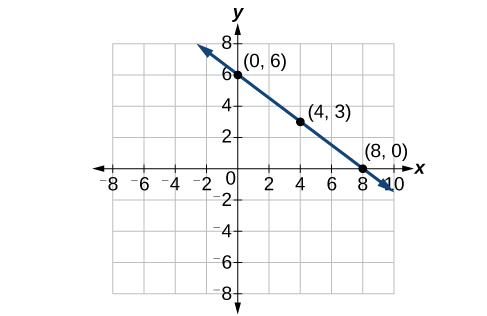# 4.1 Linear functions  (Page 7/27)

 Page 7 / 27

Is the initial value always provided in a table of values like [link] ?

No. Sometimes the initial value is provided in a table of values, but sometimes it is not. If you see an input of 0, then the initial value would be the corresponding output. If the initial value is not provided because there is no value of input on the table equal to 0, find the slope, substitute one coordinate pair and the slope into $\text{\hspace{0.17em}}f\left(x\right)=mx+b,\text{\hspace{0.17em}}$ and solve for $\text{\hspace{0.17em}}b.$

A new plant food was introduced to a young tree to test its effect on the height of the tree. [link] shows the height of the tree, in feet, $\text{\hspace{0.17em}}x\text{\hspace{0.17em}}$ months since the measurements began. Write a linear function, $\text{\hspace{0.17em}}H\left(x\right),$ where $\text{\hspace{0.17em}}x\text{\hspace{0.17em}}$ is the number of months since the start of the experiment.

 x 0 2 4 8 12 H ( x ) 12.5 13.5 14.5 16.5 18.5

$H\left(x\right)=0.5x+12.5$

## Graphing linear functions

Now that we’ve seen and interpreted graphs of linear functions, let’s take a look at how to create the graphs. There are three basic methods of graphing linear functions. The first is by plotting points and then drawing a line through the points. The second is by using the y- intercept and slope. And the third method is by using transformations of the identity function $\text{\hspace{0.17em}}f\left(x\right)=x.$

## Graphing a function by plotting points

To find points of a function, we can choose input values, evaluate the function at these input values, and calculate output values. The input values and corresponding output values form coordinate pairs. We then plot the coordinate pairs on a grid. In general, we should evaluate the function at a minimum of two inputs in order to find at least two points on the graph. For example, given the function, $\text{\hspace{0.17em}}f\left(x\right)=2x,$ we might use the input values 1 and 2. Evaluating the function for an input value of 1 yields an output value of 2, which is represented by the point $\text{\hspace{0.17em}}\left(1,2\right).\text{\hspace{0.17em}}$ Evaluating the function for an input value of 2 yields an output value of 4, which is represented by the point $\text{\hspace{0.17em}}\left(2,4\right).\text{\hspace{0.17em}}$ Choosing three points is often advisable because if all three points do not fall on the same line, we know we made an error.

Given a linear function, graph by plotting points.

1. Choose a minimum of two input values.
2. Evaluate the function at each input value.
3. Use the resulting output values to identify coordinate pairs.
4. Plot the coordinate pairs on a grid.
5. Draw a line through the points.

## Graphing by plotting points

Graph $\text{\hspace{0.17em}}f\left(x\right)=-\frac{2}{3}x+5\text{\hspace{0.17em}}$ by plotting points.

Begin by choosing input values. This function includes a fraction with a denominator of 3, so let’s choose multiples of 3 as input values. We will choose 0, 3, and 6.

Evaluate the function at each input value, and use the output value to identify coordinate pairs.

$\begin{array}{cc}\hfill x=0& \phantom{\rule{2em}{0ex}}f\left(0\right)=-\frac{2}{3}\left(0\right)+5=5⇒\left(0,5\right)\hfill \\ \hfill x=3& \phantom{\rule{2em}{0ex}}f\left(3\right)=-\frac{2}{3}\left(3\right)+5=3⇒\left(3,3\right)\hfill \\ \hfill x=6& \phantom{\rule{2em}{0ex}}f\left(6\right)=-\frac{2}{3}\left(6\right)+5=1⇒\left(6,1\right)\hfill \end{array}$

Plot the coordinate pairs and draw a line through the points. [link] represents the graph of the function $\text{\hspace{0.17em}}f\left(x\right)=-\frac{2}{3}x+5.$The graph of the linear function   f ( x ) = − 2 3 x + 5.

Graph $\text{\hspace{0.17em}}f\left(x\right)=-\frac{3}{4}x+6\text{\hspace{0.17em}}$ by plotting points.## Graphing a function using y- Intercept and slope

Another way to graph linear functions is by using specific characteristics of the function rather than plotting points. The first characteristic is its y- intercept, which is the point at which the input value is zero. To find the y- intercept, we can set $\text{\hspace{0.17em}}x=0\text{\hspace{0.17em}}$ in the equation.

the third and the seventh terms of a G.P are 81 and 16, find the first and fifth terms.
if a=3, b =4 and c=5 find the six trigonometric value sin
pls how do I factorize x⁴+x³-7x²-x+6=0
in a function the input value is called
how do I test for values on the number line
if a=4 b=4 then a+b=
a+b+2ab
Kin
commulative principle
a+b= 4+4=8
Mimi
If a=4 and b=4 then we add the value of a and b i.e a+b=4+4=8.
Tariq
what are examples of natural number
an equation for the line that goes through the point (-1,12) and has a slope of 2,3
3y=-9x+25
Ishaq
show that the set of natural numberdoes not from agroup with addition or multiplication butit forms aseni group with respect toaaddition as well as multiplication
x^20+x^15+x^10+x^5/x^2+1
evaluate each algebraic expression. 2x+×_2 if ×=5
if the ratio of the root of ax+bx+c =0, show that (m+1)^2 ac =b^2m
By the definition, is such that 0!=1.why?
(1+cosA+IsinA)(1+cosB+isinB)/(cos@+isin@)(cos$+isin$)
hatdog
Mark
jaks
Ryan
how we can draw three triangles of distinctly different shapes. All the angles will be cutt off each triangle and placed side by side with vertices touching

#### Get Jobilize Job Search Mobile App in your pocket Now!ByByBy John GabrieliBy OpenStaxBy Mary MateraBy John GabrieliBy Jemekia WeedenBy Sandhills MLTBy OpenStaxBy Mary MateraBy Gerr ZenBy OpenStax